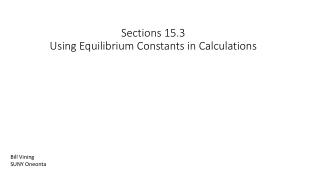DownloadDownload PresentationSections 15.3 Using Equilibrium Constants in Calculations

# Sections 15.3 Using Equilibrium Constants in Calculations

Télécharger la présentation## Sections 15.3 Using Equilibrium Constants in Calculations

- - - - - - - - - - - - - - - - - - - - - - - - - - - E N D - - - - - - - - - - - - - - - - - - - - - - - - - - -
##### Presentation Transcript

1. Sections 15.3Using Equilibrium Constants in Calculations Bill Vining SUNY Oneonta

2. Equilibrium and the Equilibrium Constant In this section… Determining an equilibrium constant Determining if a system is at equilibrium Calculating (predicting) equilibrium concentrations

3. Determining an Equilibrium Constant from Experimental Information General Idea: Write equilibrium constant expression. Measure one or more concentrations of a system at equilibrium. Use stoichiometry if needed to calculate concentrations of all species at equilibrium. Insert equilibrium values into equilibrium constant expression and calculate K.

4. Sulfur trioxide decomposes to sulfur dioxide and oxygen, If an equilibrium mixture has the following concentrations, what is the value of K? [SO3] = 0.152 M [SO2] = 0.0247 M [O2] = 0.0330 M Determining an Equilibrium Constant: Simple Example

5. An ICE table tabulates Initial concentrations, Change in concentrations, and Equilibrium concentrations Introduction to ICE Tables: Determining K with less data. Nitrogen and hydrogen form ammonia. Initial concentrations of the reactants are [N2] = 0.1000 M and [H2] = 0.2200 M. After the system reaches equilibrium, the nitrogen concentration has decreased to 0.0271 M. Initial (M) Change (M) Equilibrium (M)

6. Nitrogen and hydrogen form ammonia. Initial concentrations of the reactants are [N2] = 0.1000 M and [H2] = 0.2200 M. After the system reaches equilibrium, the nitrogen concentration has decreased to 0.0271 M. Use x to determine equilibrium concentrations. Initial (M) 0.1000 0.2200 0 Change (M) -x -3x +2x Equilibrium (M) 0.1000-x 0.2200-3x 2x Determine the value of x. Use equilibrium concentrations to calculate K:

7. The reaction quotient, Q, has the same form as the equilibrium constant expression, • but uses actual concentrations, whether they are at equilibrium or not. • How Q is used: • Write equilibrium constant expression. • Insert concentrations and calculate Q. • If Q = K, the system is at equilibrium. • If Q > K, there are too many products and the system will “shift left” to form more reactants. • If Q < K, there are not enough products and the system will “shift right,” forming more products. Determining if a System is at Equilibrium: Q, the Reaction Quotient

8. The following system has K = 22.3 at a particular temperature. If the concentrations are as given below, is the system at equilibrium? If not, in which direction will the system react to reach equilibrium? [Cl2] = 0.300 M [F2] = 0.620 M [ClF] = 0.120 M Determining if a System is at Equilibrium: Example using Q

9. Predicting Equilibrium Concentrations for a System Moving to Equilibrium • In which direction will it react to attain equilibrium? • What will the concentrations be once equilibrium is reached? • How this is done: • Write equilibrium constant expression. • Insert concentrations and calculate Q. • If Q = K, the system is at equilibrium. • If Q > K, there are too many products and the system will “shift left” to form more reactants. • If Q < K, there are not enough products and the system will “shift right,” forming more products. • Set up an ICE table and determine equilibrium concentrations in terms of initial concentrations and x. • Insert these into the equilibrium constant expression and solve to get the numerical value of x. • Use x and initial concentrations to determine equilibrium concentrations.

10. Consider the following system, where butane isomerizes to form isobutene. A flask initially contains 0.200 M butane. What will the concentrations of butane and isobutene be when equilibrium is reached? Reaction will shift to the right, forming isobutene. Set up an ICE table: Predicting Equilibrium Concentrations: Example [butane] [isobutene] Initial (M) Change (M) Equilibrium (M)

11. Consider the following system, where butane isomerizes to form isobutene. Predicting Equilibrium Concentrations: Example Solve for x: [butane] [isobutene] Initial (M) 0.200 0 Change (M) -x +x Equilibrium (M) 0.200 – x x Determine equilibrium concentrations: Insert concentration equations into equilibrium expression: Do a final check of the answers: